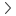# Pip Calculator

Traders' toolsPip calculator

0

Synthetic

Financial

### How to calculate pip value

The pip value for a contract on DMT5 is calculated based on this formula:

Pip value = point value × volume × contract size

For synthetic accounts, the pip value is calculated in USD.

For financial accounts, the pip value is in the quote currency for forex pairs.

### Example calculation

Let’s say you want to trade 1 lot of Volatility 75 Index.1. The point value is derived from the current digits of the assets. In the example, the digit is 2, so the point value is 0.01.
2. The contract size is one standard lot of Volatility 75 index = 1

So your pip value is 0.01 USD.

Let’s say you want to trade 2 lots of EUR/USD.1. The point value is derived from the current digits of the assets. In the example, the digit is 5, so the point value is 0.00001.
2. One standard lot of forex = 100,000 units

So your pip value is 2 USD.